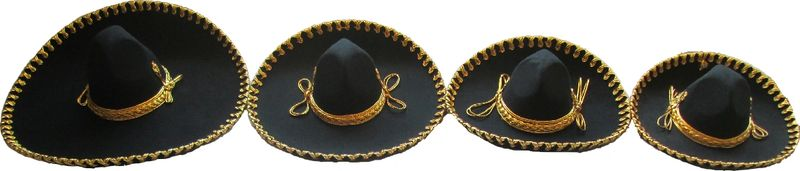Can't figure out what size you need?

Hat Size/ Medida de Sombrero:
Shoe Sizes/ Medida de Zapato:Dresses: (shirt size)
T-4 through T-14 = little girl sizes
T-32 through T-34 = women's "small"
T-36 through T-38 = women's "medium"
T-40 through T-42 = women's "large"
T-44 = women's "ex-large"

Skirts: (length from waist down)
50 cm- 105 cm

Men's Shirts:
T-4 through T-18 = little boy size (usually fits ages 4- 12)
T-32 through T-34 = men's "small"
T-36 = men's "medium"
T-38 through T-40 = men's "large"
T-42 through T-44 = men's "ex-large"

Men's Pants:
T-4 =
T-6 =
T-8 =
T-10 =
T-12 =
T-14 =
T-16 =
T-28 =
T-30 =
T-32 =
T-34 =
T-36 =
T-38 =
T-40 =
T-42 =
T-44 =